Like   Tweet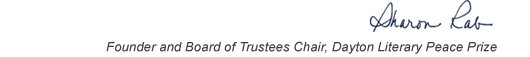table div table+table+table+table div table{width:100%;padding:0}table div table+table+table+table div table img{width:96.23%;padding:0;float:none}table div table+table+table+table div table td{width:100%;padding:0 1.88% 18px}/* styles */This list includes the winners and runners-up for 2019 and we have rich background information on each one on our website.

▪ Under Past Winners. scroll down to the year and click on it.
▪ You will have the option of clicking on a winner, a runner-up, or the finalists.
▪ If you click on the photo of winners and runners-up, you will find the author’s photo and bio, a selection from the book, the judges’ citation, the writer’s reflection on literature and peace, and the option of watching the introduction and acceptance speech.
 ▪ Under Past Winners. scroll down to the year and click on it.
 ▪ You will have the option of clicking on a winner, a runner-up, or the finalists.
 ▪ If you click on the photo of winners and runners-up, you will find the author’s photo and bio, a selection from the book, the judges’ citation, the writer’s reflection on literature and peace, and the option of watching the introduction and acceptance speech.

On the bottom right of the home page, you can click on COREScholar, which is built by the Chair of our Curriculum Committee Carol Loranger, Associate Dean of the College of Liberal Arts at Wright State, and maintained for us by Wright State University. There you can find interviews, TED Talks, articles, other books, films, etc. by and about each of our winning and runner-up authors. It is a great resource for students, book clubs, and readers who would like to explore an author in depth.

 table div table+table+table+table+table+table div table{width:100%;padding:0}table div table+table+table+table+table+table div table img{width:96.23%;padding:0;float:none}table div table+table+table+table+table+table div table td{width:100%;padding:0 1.88% 18px}/* styles */# Our Finalists - Fiction

 table div table+table+table+table+table+table+table+table div table{width:100%;padding:0}table div table+table+table+table+table+table+table+table div table img{width:96.23%;padding:0;float:none}table div table+table+table+table+table+table+table+table div table td{width:100%;padding:0 1.88% 18px}/* styles */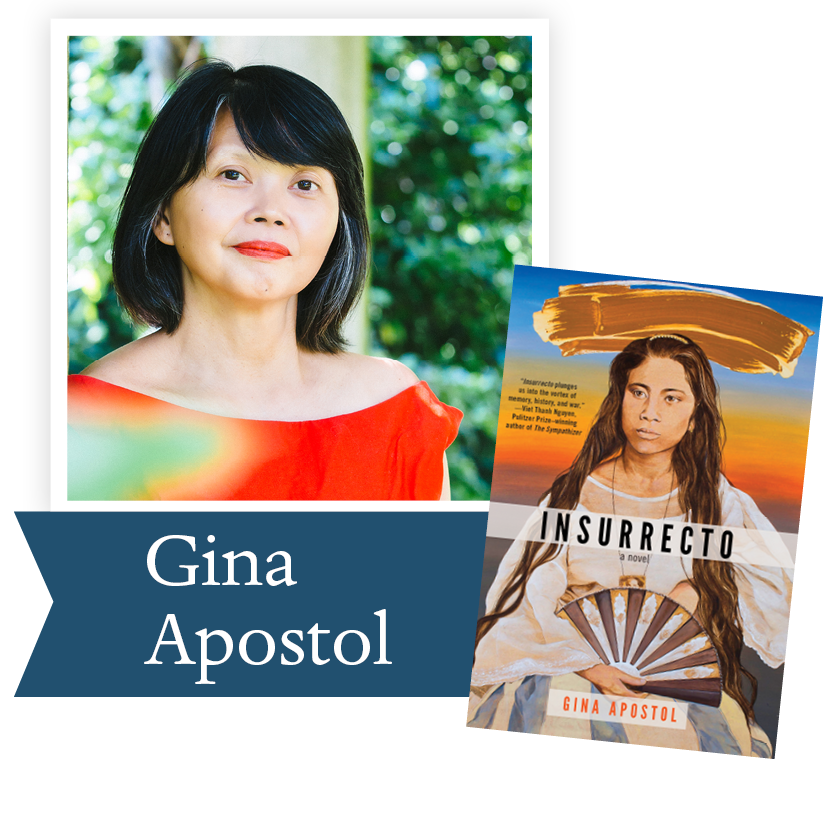Insurrecto by Gina Apostol, Soho Press: Histories and personalities collide in this literary tour-de-force about the Philippines’ present and America’s past. Two women, a Filipino translator and an American filmmaker, go on a road trip in Duterte’s Philippines, collaborating and clashing in the writing of a film script about a massacre during the Philippine-American War. Insurrecto contains within its dramatic action two rival scripts—one about a white photographer, the other about a Filipino school teacher.

 table div table+table+table+table+table+table+table+table+table+table div table{width:100%;padding:0}table div table+table+table+table+table+table+table+table+table+table div table img{width:96.23%;padding:0;float:none}table div table+table+table+table+table+table+table+table+table+table div table td{width:100%;padding:0 1.88% 18px}/* styles */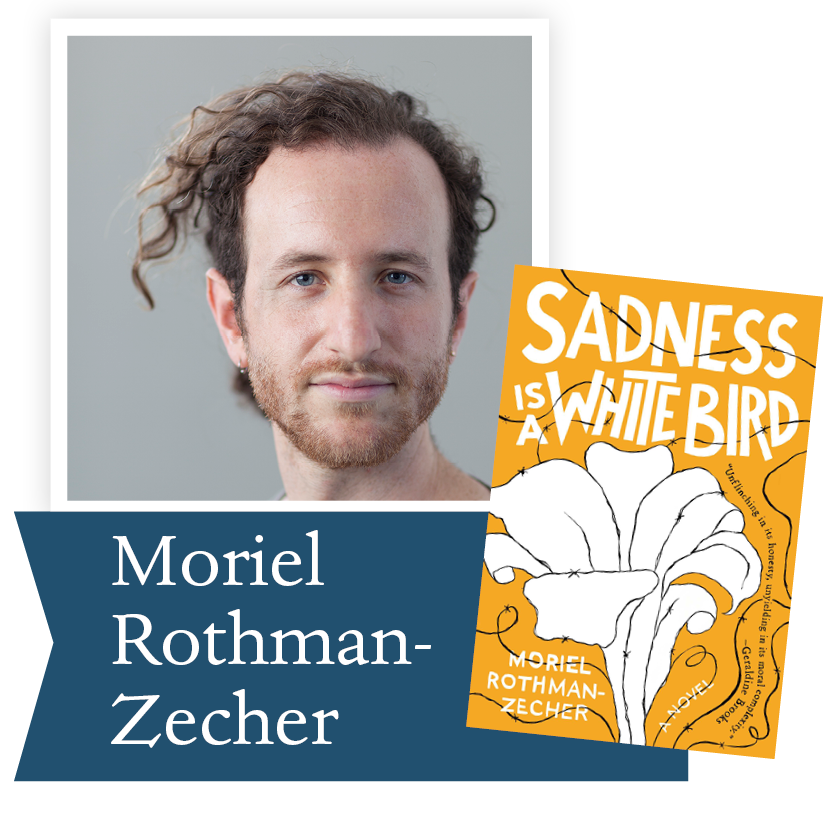Sadness Is A White Bird by Moriel Rothman-Zecher, Atria Books (S&S): In this debut novel from the MacDowell Colony fellow and National Book Foundation “5 Under 35” honoree, a young man prepares to serve in the Israeli army while also trying to reconcile his close relationship to two Palestinian siblings with his deeply ingrained loyalties to family and country. Powerful, important, and timely, Sadness Is A White Bird explores one man’s attempts to find a place for himself, discovering in the process a beautiful, against-the-odds love in the darkness of a never-ending conflict.

 table div table+table+table+table+table+table+table+table+table+table+table+table div table{width:100%;padding:0}table div table+table+table+table+table+table+table+table+table+table+table+table div table img{width:96.23%;padding:0;float:none}table div table+table+table+table+table+table+table+table+table+table+table+table div table td{width:100%;padding:0 1.88% 18px}/* styles */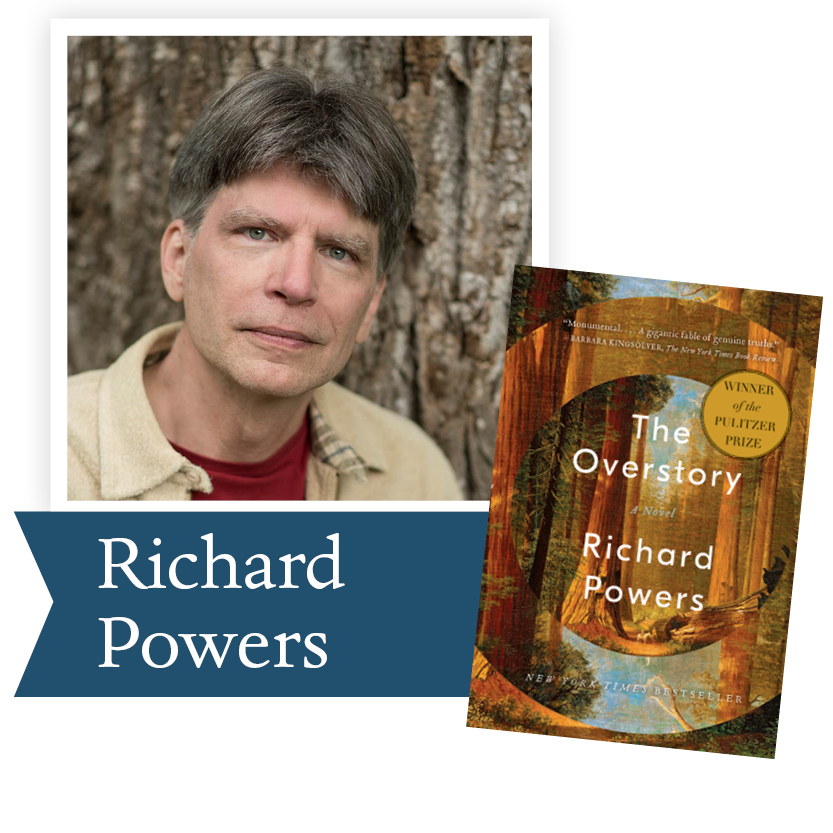The Overstory by Richard Powers, WW Norton & Co: Winner of the 2019 Pulitzer Prize in Fiction, The Overstory is a sweeping, impassioned work of activism and resistance that is also a stunning evocation of—and paean to—the natural world. There is a world alongside ours—vast, slow, interconnected, resourceful, magnificently inventive, and almost invisible to us. This is the story of a handful of people who learn how to see that world and are drawn into its unfolding catastrophe.

 table div table+table+table+table+table+table+table+table+table+table+table+table+table+table div table{width:100%;padding:0}table div table+table+table+table+table+table+table+table+table+table+table+table+table+table div table img{width:96.23%;padding:0;float:none}table div table+table+table+table+table+table+table+table+table+table+table+table+table+table div table td{width:100%;padding:0 1.88% 18px}/* styles */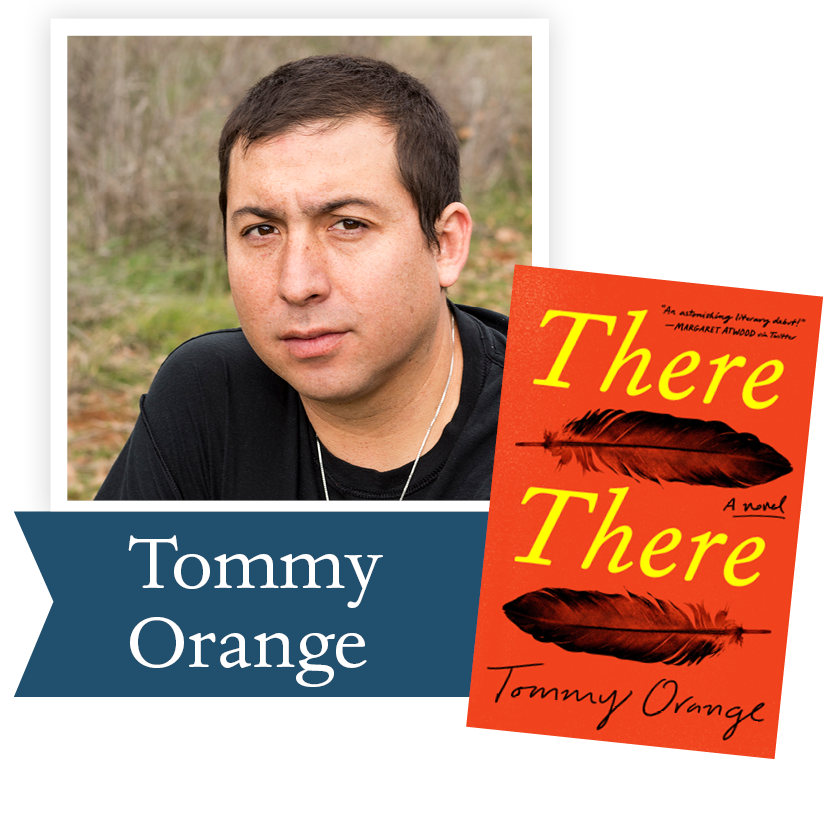There There by Tommy Orange, Knopf: Fierce, funny, suspenseful, and thoroughly modern, There There offers a kaleidoscopic look at Native American life in Oakland, California. Writing in a voice full of poetry and rage, exploding onto the page with urgency and force, Tommy Orange has created a stunning novel that grapples with a complex and painful history, with an inheritance of beauty and profound spirituality, and with a plague of addiction, abuse, and suicide.

 table div table+table+table+table+table+table+table+table+table+table+table+table+table+table+table+table div table{width:100%;padding:0}table div table+table+table+table+table+table+table+table+table+table+table+table+table+table+table+table div table img{width:96.23%;padding:0;float:none}table div table+table+table+table+table+table+table+table+table+table+table+table+table+table+table+table div table td{width:100%;padding:0 1.88% 18px}/* styles */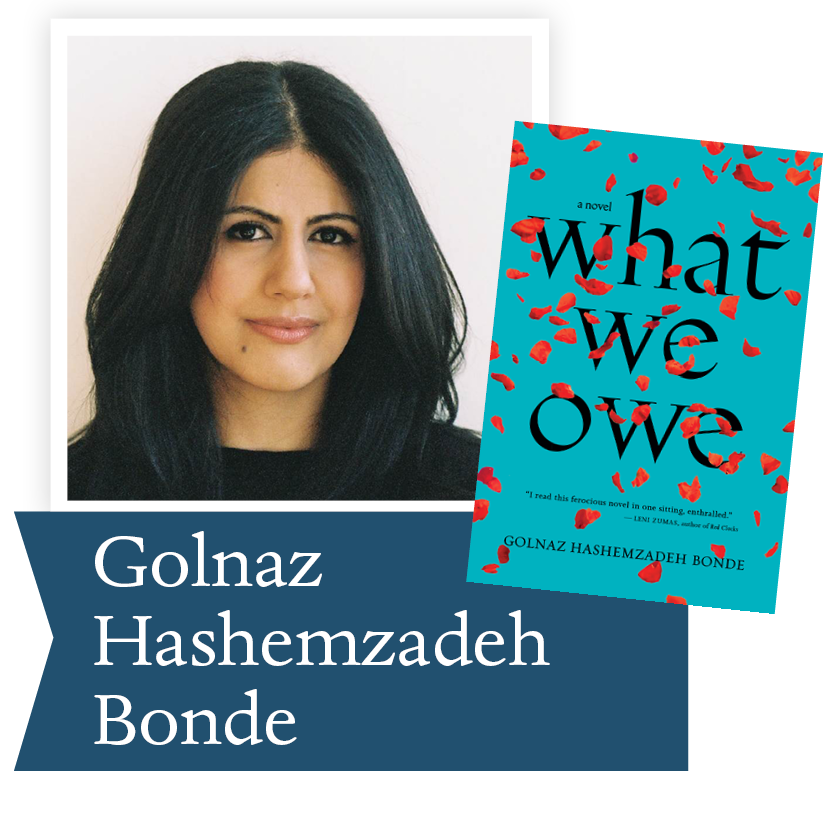What We Owe by Golnaz Hashemzadeh Bonde, Houghton, Mifflin, Harcourt: Here is an extraordinary story of exile, dislocation, and the emotional minefields between mothers and daughters; a story of love, guilt and dreams for a better future, vibrating with both sorrow and an unquenchable joie de vivre. With its startling honesty, dark wit, and irresistible momentum, What We Owe introduces a fierce and necessary new voice in international fiction.

 table div table+table+table+table+table+table+table+table+table+table+table+table+table+table+table+table+table+table div table{width:100%;padding:0}table div table+table+table+table+table+table+table+table+table+table+table+table+table+table+table+table+table+table div table img{width:96.23%;padding:0;float:none}table div table+table+table+table+table+table+table+table+table+table+table+table+table+table+table+table+table+table div table td{width:100%;padding:0 1.88% 18px}/* styles */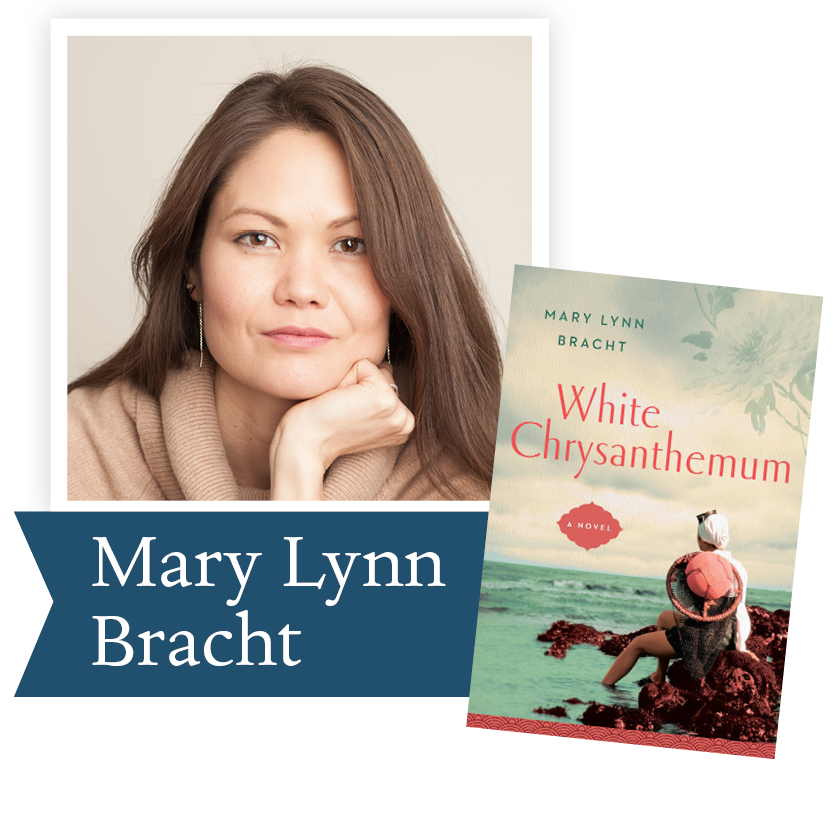White Chrysanthemum by Mary Lynn Bracht, GP Putnam’s Sons, PRH: White Chrysanthemum brings to life the heartbreaking history of Korea through the deeply moving and redemptive story of two sisters separated by World War II. It is a moving fictional account of a shockingly pervasive real-life assault—the sexual slavery of an estimated 200,000 Korean women during the Second World War.

 table div table+table+table+table+table+table+table+table+table+table+table+table+table+table+table+table+table+table+table+table div table{width:100%;padding:0}table div table+table+table+table+table+table+table+table+table+table+table+table+table+table+table+table+table+table+table+table div table img{width:96.23%;padding:0;float:none}table div table+table+table+table+table+table+table+table+table+table+table+table+table+table+table+table+table+table+table+table div table td{width:100%;padding:0 1.88% 18px}/* styles */# Our Finalists - Nonfiction

 table div table+table+table+table+table+table+table+table+table+table+table+table+table+table+table+table+table+table+table+table+table+table div table{width:100%;padding:0}table div table+table+table+table+table+table+table+table+table+table+table+table+table+table+table+table+table+table+table+table+table+table div table img{width:96.23%;padding:0;float:none}table div table+table+table+table+table+table+table+table+table+table+table+table+table+table+table+table+table+table+table+table+table+table div table td{width:100%;padding:0 1.88% 18px}/* styles */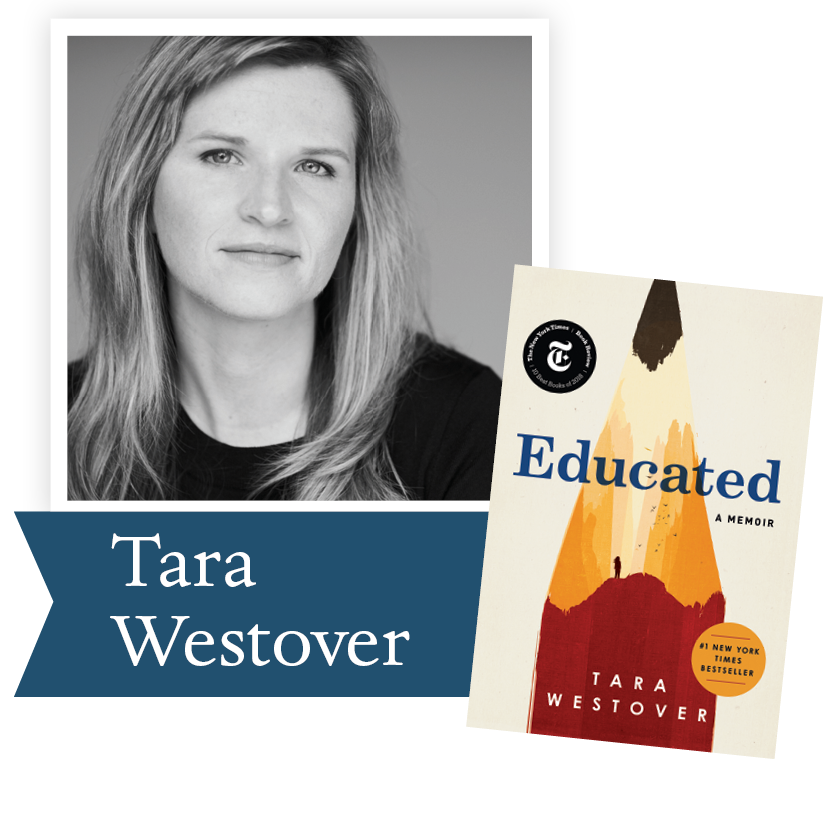Educated by Tara Westover, Random House: With the acute insight that distinguishes all great writers, Westover has crafted a universal coming-of-age story that gets to the heart of what an education offers: the perspective to see one's life through new eyes, and the will to change it.

 table div table+table+table+table+table+table+table+table+table+table+table+table+table+table+table+table+table+table+table+table+table+table+table+table div table{width:100%;padding:0}table div table+table+table+table+table+table+table+table+table+table+table+table+table+table+table+table+table+table+table+table+table+table+table+table div table img{width:96.23%;padding:0;float:none}table div table+table+table+table+table+table+table+table+table+table+table+table+table+table+table+table+table+table+table+table+table+table+table+table div table td{width:100%;padding:0 1.88% 18px}/* styles */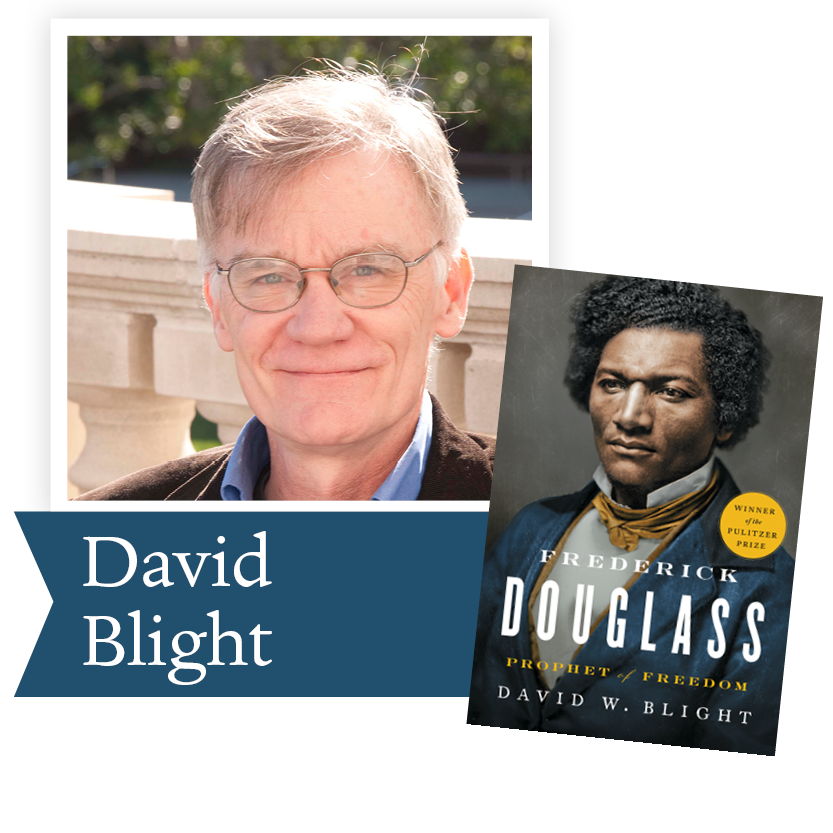Frederick Douglass by David Blight, Simon and Schuster: In his “cinematic and deeply engaging” (The New York Times Book Review) biography, Blight has drawn on new information held in a private collection that few other historians have consulted, as well as recently discovered issues of Douglass’s newspapers. Blight’s Pulitzer Prize-winning book tells the fascinating story of Douglass’s two marriages and his complex extended family.

 table div table+table+table+table+table+table+table+table+table+table+table+table+table+table+table+table+table+table+table+table+table+table+table+table+table+table div table{width:100%;padding:0}table div table+table+table+table+table+table+table+table+table+table+table+table+table+table+table+table+table+table+table+table+table+table+table+table+table+table div table img{width:96.23%;padding:0;float:none}table div table+table+table+table+table+table+table+table+table+table+table+table+table+table+table+table+table+table+table+table+table+table+table+table+table+table div table td{width:100%;padding:0 1.88% 18px}/* styles */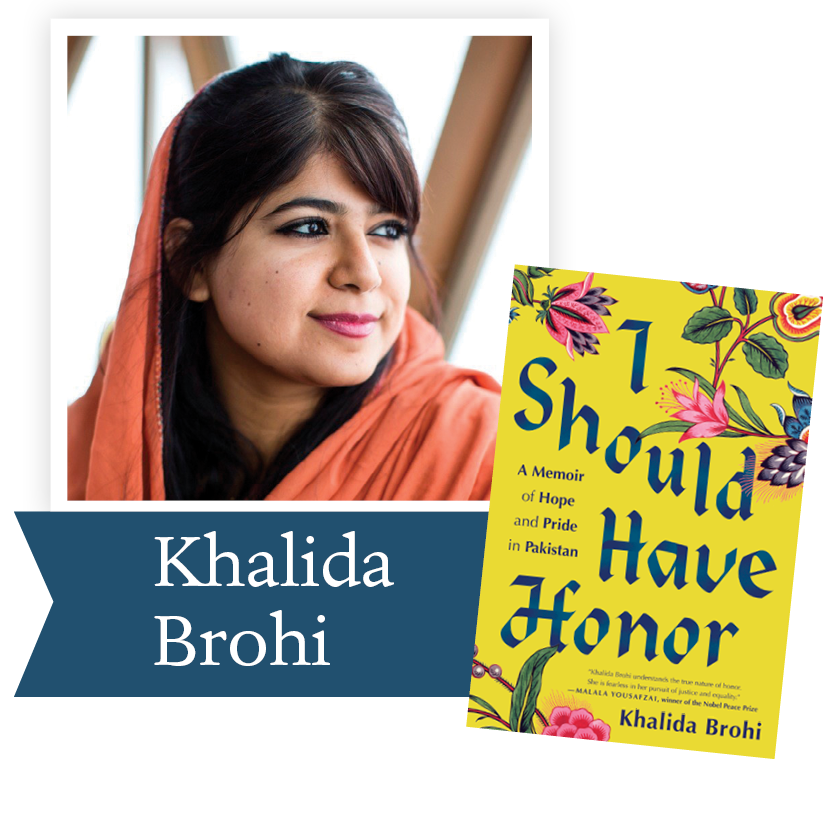I Should Have Honor by Khalida Brohi, Random House: A fearless memoir about tribal life in Pakistan—and the act of violence that inspired one ambitious young woman to pursue a life of activism and female empowerment. And ultimately, she learned that the only way to eradicate the parts of a culture she despised was to fully embrace the parts of it that she loved.

 table div table+table+table+table+table+table+table+table+table+table+table+table+table+table+table+table+table+table+table+table+table+table+table+table+table+table+table+table div table{width:100%;padding:0}table div table+table+table+table+table+table+table+table+table+table+table+table+table+table+table+table+table+table+table+table+table+table+table+table+table+table+table+table div table img{width:96.23%;padding:0;float:none}table div table+table+table+table+table+table+table+table+table+table+table+table+table+table+table+table+table+table+table+table+table+table+table+table+table+table+table+table div table td{width:100%;padding:0 1.88% 18px}/* styles */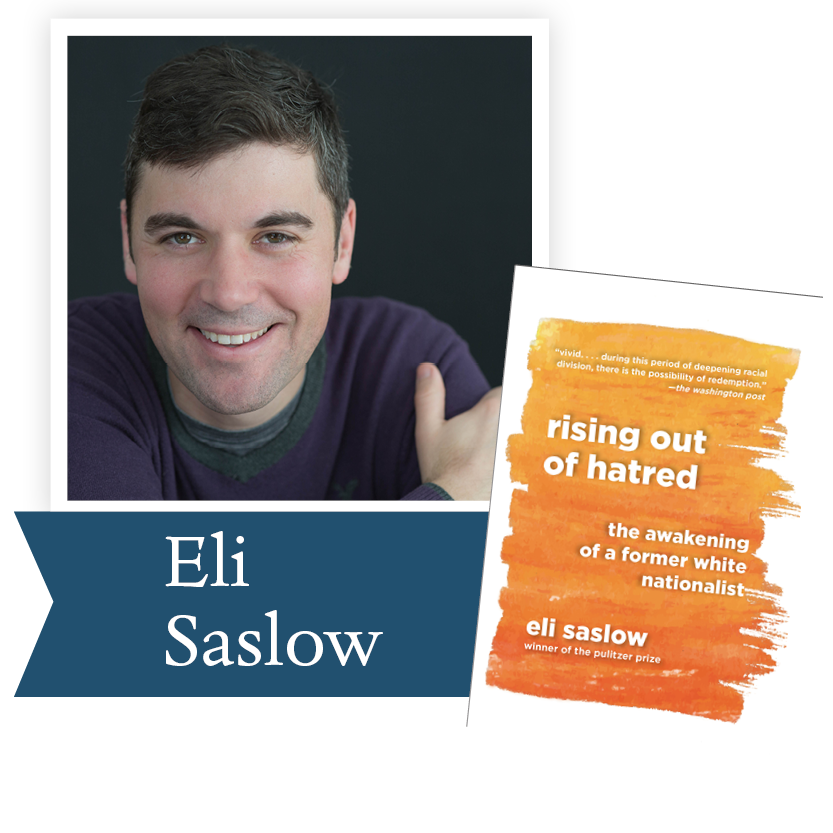Rising Out of Hatred by Eli Saslow, Doubleday: From a Pulitzer Prize-winning reporter, the powerful story of how a prominent white supremacist changed his heart and mind. With great empathy and narrative verve, Eli Saslow asks what Derek's story can tell us about America's increasingly divided nature. This is a book to help us understand the American moment and to help us better understand one another.

 table div table+table+table+table+table+table+table+table+table+table+table+table+table+table+table+table+table+table+table+table+table+table+table+table+table+table+table+table+table+table div table{width:100%;padding:0}table div table+table+table+table+table+table+table+table+table+table+table+table+table+table+table+table+table+table+table+table+table+table+table+table+table+table+table+table+table+table div table img{width:96.23%;padding:0;float:none}table div table+table+table+table+table+table+table+table+table+table+table+table+table+table+table+table+table+table+table+table+table+table+table+table+table+table+table+table+table+table div table td{width:100%;padding:0 1.88% 18px}/* styles */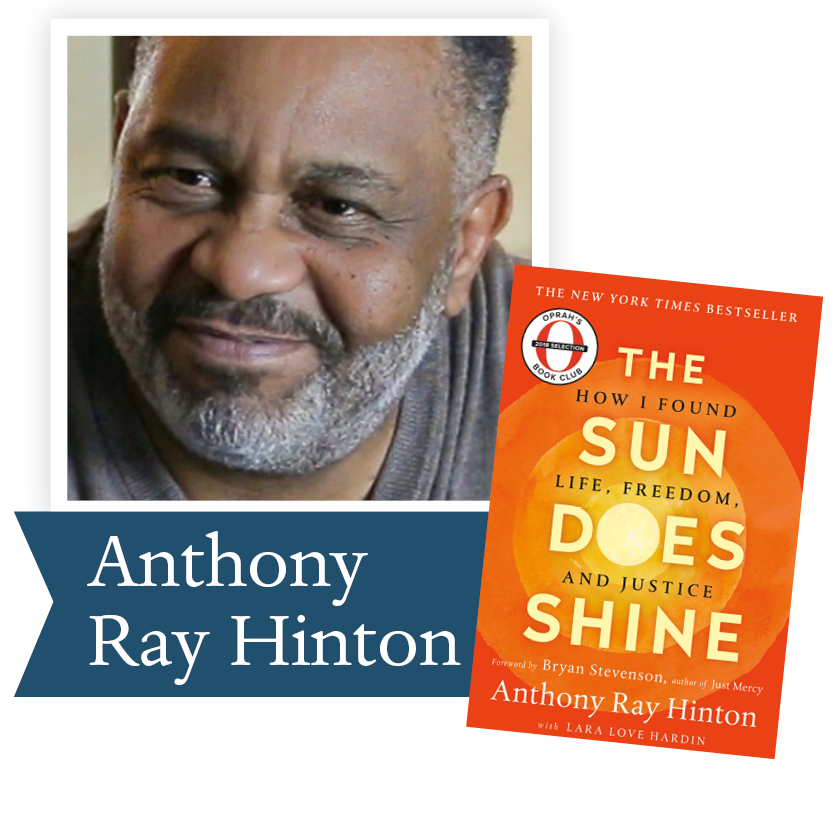The Sun Does Shine: How I Found Life and Freedom on Death Row by Anthony Ray Hinton with Laura Love Hardin, St. Martins: With a foreword by Bryan Stevenson, The Sun Does Shine is an extraordinary testament to the power of hope sustained through the darkest times. Destined to be a classic memoir of wrongful imprisonment and freedom won, The Sun Does Shine tells Hinton’s dramatic 30-year journey and shows how you can take away a man’s freedom, but you can’t take away his imagination, humor, or joy.

 table div table+table+table+table+table+table+table+table+table+table+table+table+table+table+table+table+table+table+table+table+table+table+table+table+table+table+table+table+table+table+table+table div table{width:100%;padding:0}table div table+table+table+table+table+table+table+table+table+table+table+table+table+table+table+table+table+table+table+table+table+table+table+table+table+table+table+table+table+table+table+table div table img{width:96.23%;padding:0;float:none}table div table+table+table+table+table+table+table+table+table+table+table+table+table+table+table+table+table+table+table+table+table+table+table+table+table+table+table+table+table+table+table+table div table td{width:100%;padding:0 1.88% 18px}/* styles */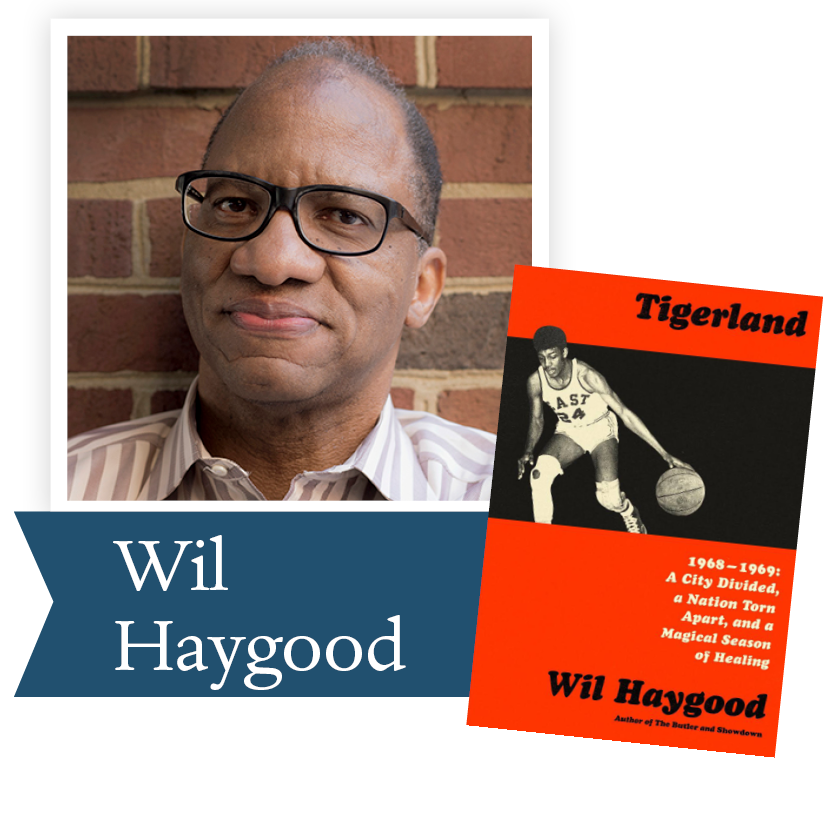Tigerland by Wil Haygood, Knopf: From the author of the best-selling The Butler–an emotional, inspiring story of two teams from a poor, black, segregated high school in Ohio, who, in the midst of the racial turbulence of 1968/1969, win the Ohio state baseball and basketball championships in the same year.

 table div table+table+table+table+table+table+table+table+table+table+table+table+table+table+table+table+table+table+table+table+table+table+table+table+table+table+table+table+table+table+table+table+table+table div table{width:100%;padding:0}table div table+table+table+table+table+table+table+table+table+table+table+table+table+table+table+table+table+table+table+table+table+table+table+table+table+table+table+table+table+table+table+table+table+table div table img{width:96.23%;padding:0;float:none}table div table+table+table+table+table+table+table+table+table+table+table+table+table+table+table+table+table+table+table+table+table+table+table+table+table+table+table+table+table+table+table+table+table+table div table td{width:100%;padding:0 1.88% 18px}/* styles */## This week's featured Holbrooke author

This newsletter’s suggestion comes from a writer for National Catholic Register, Jason Craig, who recommends novels by our 2013 Ambassador Richard C. Holbrooke Lifetime Achievement Winner, Wendell Berry.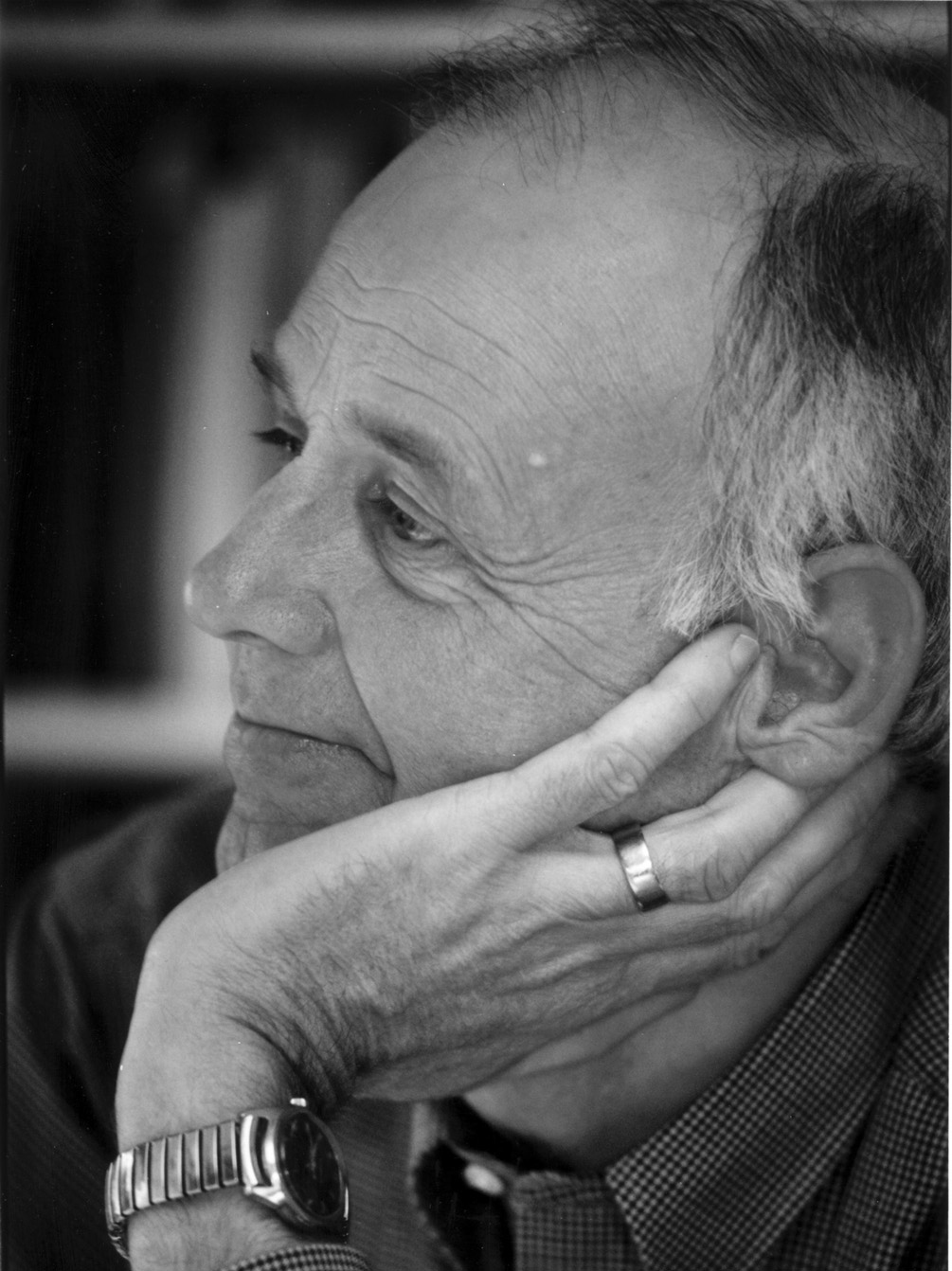By becoming unbound in the name of freedom we’ve become divorced from all sorts of things — most notably, each other.
Jason Craig

So here are some ideas for reading in the genre of “things that are really making us sick,” with a special eye toward social isolation, a thing we were experiencing long before any virus:

I don’t read enough novels, but Jayber Crow by Wendell Berry is one that I’ll pick up over and over. Berry is known for various reasons – poetry, essays, novels – but if he is known, it is known he writes from a quintessentially agrarian point of view. He watched firsthand his own farm-based community unravel in the wake of economic and technological changes, and he has painfully shown us in books like The Unsettling of America what we really lost when we lost our local cultures.

Some dismiss such views with a sort of flippancy (“What, you don’t like cheap tomatoes available whenever you want some?”), but anyone familiar with Berry’s work knows his penetrating and realistic observations are anything but romanticism. With the loss of local cultures, we lost more than our agrarian backbones and strong hands, because by becoming unbound in the name of freedom we’ve become divorced from all sorts of things — most notably, each other.

Jayber Crow follows Jayber (not his real name), an essentially orphaned boy, as he moves from belonging to disorientation, from the pseudo-freedom a young man thinks he wants to re-finding home, place and people. As I’ve written elsewhere, we can think of Jayber as a sort of priest, since he eventually makes an offering of himself for love that even results in a voluntary celibacy. He cleans the local church and even digs the graves for the dead. His main work of barbering, as one of his customers puts it, is not really a “line of work” but more of a position held, a vocation. In a time when belonging and place are things we simply don’t possess, and work is a means of paying bills but void of meaning, Jayber Crow is a potent read. In a time of social distancing, this novel will remind us how important the people near us really are. (If you wanted a Berry novel that is a bit shorter, however, you can grab Hannah Coulter, which writes of the same community but from a different lens, and it’s a bit shorter.)

 table div table+table+table+table+table+table+table+table+table+table+table+table+table+table+table+table+table+table+table+table+table+table+table+table+table+table+table+table+table+table+table+table+table+table+table+table+table div table{width:100%;padding:0}table div table+table+table+table+table+table+table+table+table+table+table+table+table+table+table+table+table+table+table+table+table+table+table+table+table+table+table+table+table+table+table+table+table+table+table+table+table div table img{width:96.23%;padding:0;float:none}table div table+table+table+table+table+table+table+table+table+table+table+table+table+table+table+table+table+table+table+table+table+table+table+table+table+table+table+table+table+table+table+table+table+table+table+table+table div table td{width:100%;padding:0 1.88% 18px}/* styles */table div table+table+table+table+table+table+table+table+table+table+table+table+table+table+table+table+table+table+table+table+table+table+table+table+table+table+table+table+table+table+table+table+table+table+table+table+table+table+table div table{width:100%;padding:0}table div table+table+table+table+table+table+table+table+table+table+table+table+table+table+table+table+table+table+table+table+table+table+table+table+table+table+table+table+table+table+table+table+table+table+table+table+table+table+table div table img{width:96.23%;padding:0;float:none}table div table+table+table+table+table+table+table+table+table+table+table+table+table+table+table+table+table+table+table+table+table+table+table+table+table+table+table+table+table+table+table+table+table+table+table+table+table+table+table div table td{width:100%;padding:0 1.88% 18px}/* styles */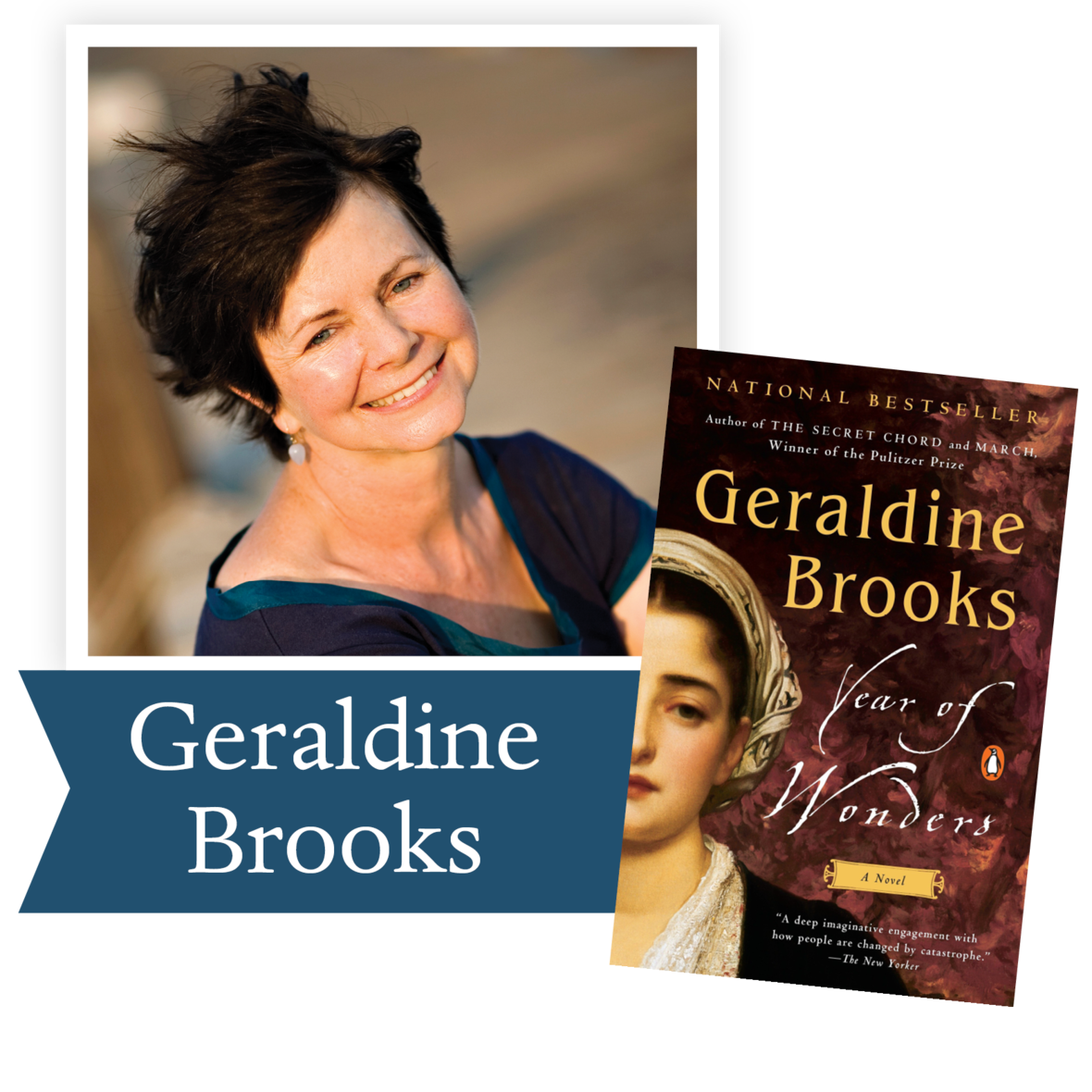“Plague stories remind us that we cannot manage without community . . . Year of Wonders is a testament to that very notion.” – The Washington Post

An unforgettable tale, set in 17th century England, of a village that quarantines itself to arrest the spread of the plague, from the author The Secret Chord and of March, winner of the Pulitzer Prize.

Ron Rollins, Dayton Daily News Ideas and Voices Editor, will moderate the discussion of The Year of Wonders.

Email Emily Kretzer to be added to the list. We'll send details as they become available.

 table div table+table+table+table+table+table+table+table+table+table+table+table+table+table+table+table+table+table+table+table+table+table+table+table+table+table+table+table+table+table+table+table+table+table+table+table+table+table+table+table+table div table{width:100%;padding:0}table div table+table+table+table+table+table+table+table+table+table+table+table+table+table+table+table+table+table+table+table+table+table+table+table+table+table+table+table+table+table+table+table+table+table+table+table+table+table+table+table+table div table img{width:96.23%;padding:0;float:none}table div table+table+table+table+table+table+table+table+table+table+table+table+table+table+table+table+table+table+table+table+table+table+table+table+table+table+table+table+table+table+table+table+table+table+table+table+table+table+table+table+table div table td{width:100%;padding:0 1.88% 18px}/* styles */Support the Dayton Literary Peace Prize when you shop with Amazon using this link.

 table div table+table+table+table+table+table+table+table+table+table+table+table+table+table+table+table+table+table+table+table+table+table+table+table+table+table+table+table+table+table+table+table+table+table+table+table+table+table+table+table+table+table+table div table{width:100%;padding:0}table div table+table+table+table+table+table+table+table+table+table+table+table+table+table+table+table+table+table+table+table+table+table+table+table+table+table+table+table+table+table+table+table+table+table+table+table+table+table+table+table+table+table+table div table img{width:96.23%;padding:0;float:none}table div table+table+table+table+table+table+table+table+table+table+table+table+table+table+table+table+table+table+table+table+table+table+table+table+table+table+table+table+table+table+table+table+table+table+table+table+table+table+table+table+table+table+table div table td{width:100%;padding:0 1.88% 18px}/* styles */